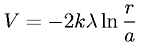Equations > Physics > Electricity and Magnetism > Potential due to a line charge

### Potential due to a line chargeLatex Code:

MathML Code:

 $V=-2k\lambda \mathrm{ln}\fracra$

MathType 5.0: